# Point Groups 2 (Worksheet)

Name: ______________________________

Section: _____________________________

Student ID#:__________________________

Work in groups on these problems. You should try to answer the questions without referring to your textbook. If you get stuck, try asking another group for help.

Determine the point group assignment for the following: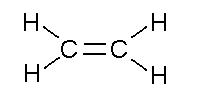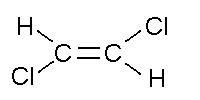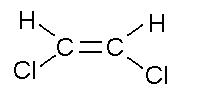acetylene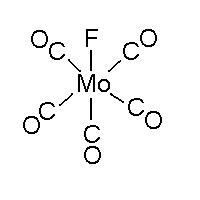$$PtCl_2I_2^{2-}$$ (cis and trans) $$XeOF_4$$ $$AsF_5$$ $$PF_3$$ $$SCl_4$$ benzene $$BrF_3$$ $$S_8$$ (crown) Staggered ethane $$I_3^-$$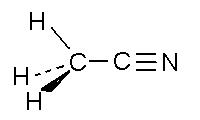$$Mo(CO)_6$$ A black cat at its highest symmetry $$CS_2$$ A three-legged stool $$PFCl_2$$ A rectangular brick